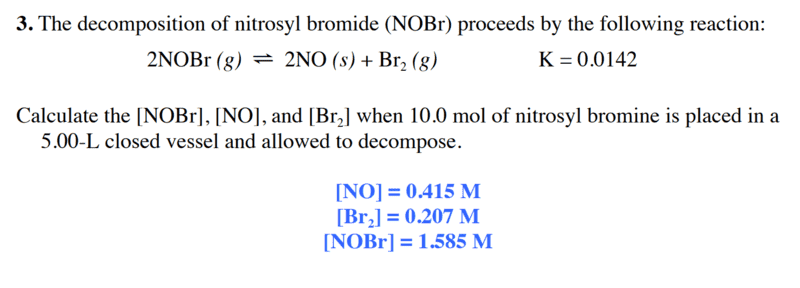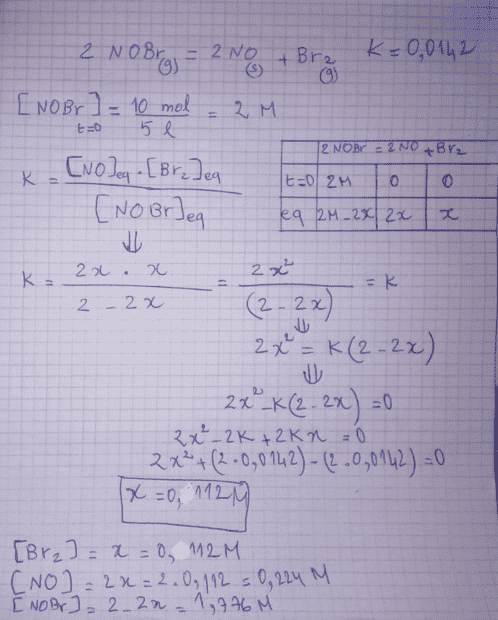# An exercise about chemical equilibrium

• Chemistry
• samy4408

#### samy4408

Homework Statement
my answer is an image in the post
Relevant Equations
no particular equations
Hello i am trying to solve a problem set about chemical equilibrium , the issue is that my results don't correspond to the correction . can someone tell me what is wrong with my answer , thanks
here is the problem and his correction :$$k = \frac{[NO]^2[Br_2]}{[NOBr]^2}$$
•